Home > CC4 > Chapter A > Lesson A.1.3 > ProblemA-32

A-32.
1. Read the Math Notes box in this lesson. Evaluate the expressions below for the given values of x and y. Homework Help ✎

1.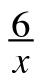+ 9 if x = 3

2. x2 + y if x = 2 and y = 1

3. −2xy if x = 5 and y = −3

4. 2x2y if x = −3 and y = 8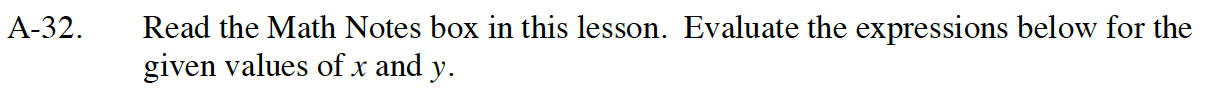Rewrite the expression with the number in place of the variable and simplify if possible.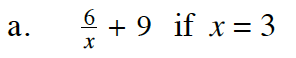11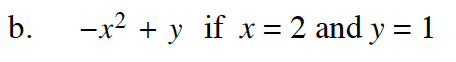−(2)² + 1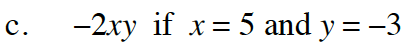−2(5)(−3) = −10(−3)

30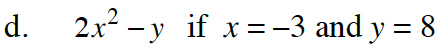2(−3)² − (8)